Successfully reported this slideshow.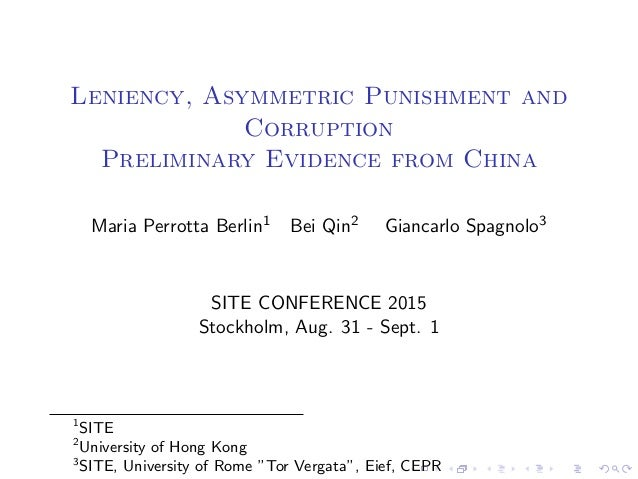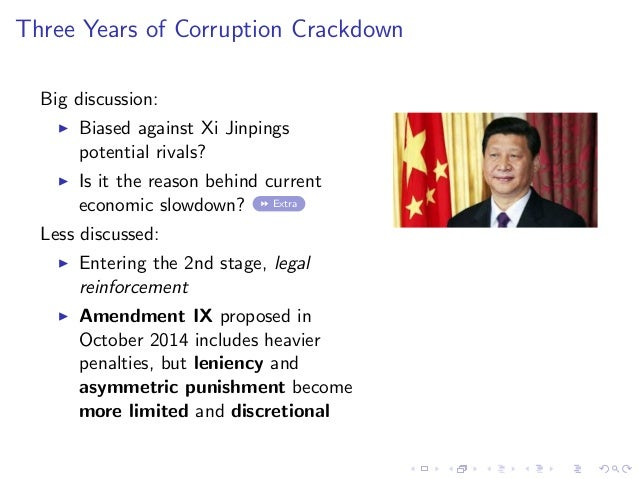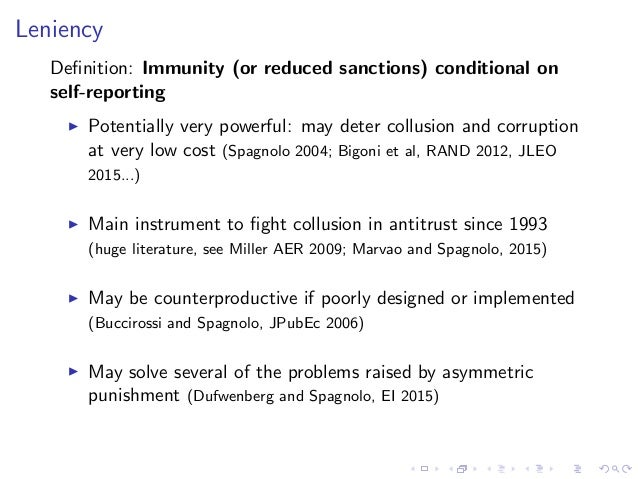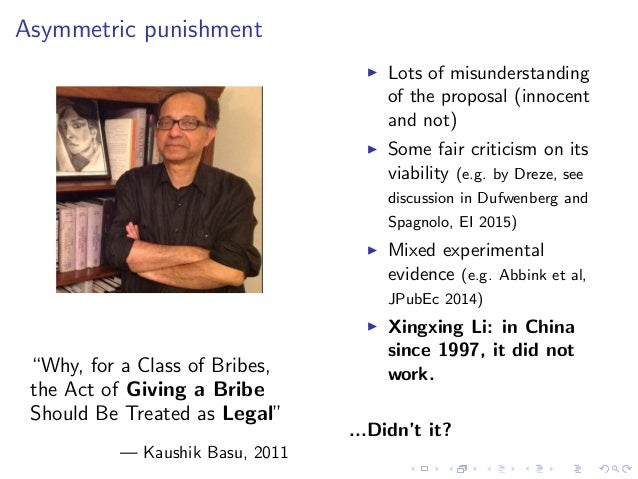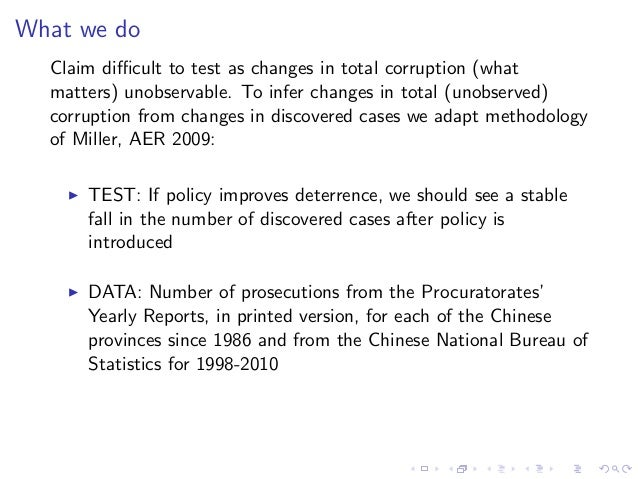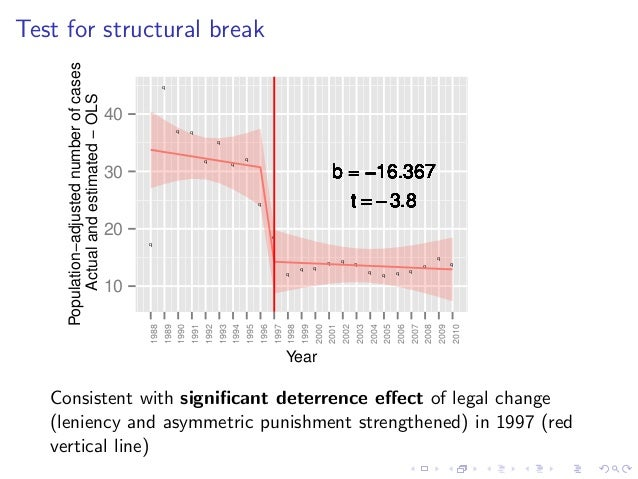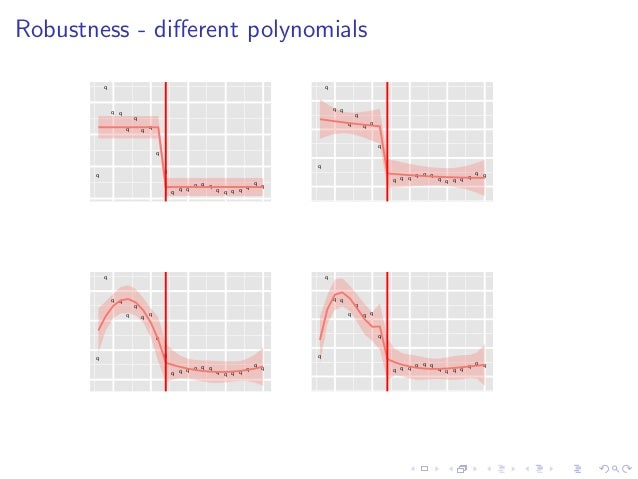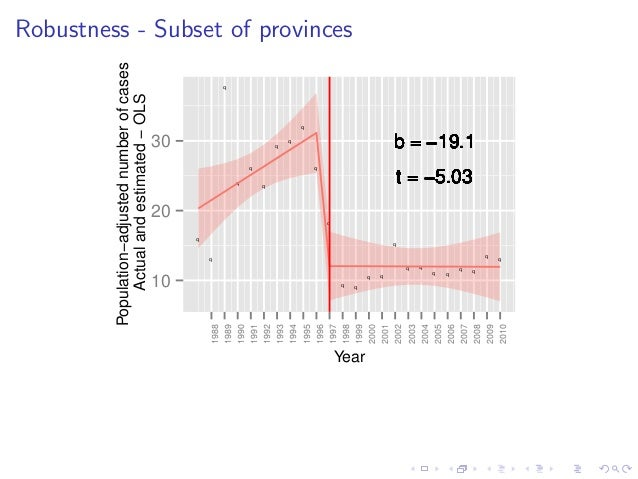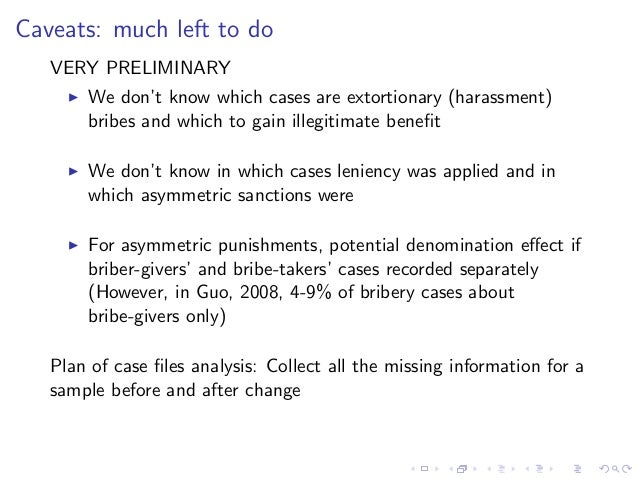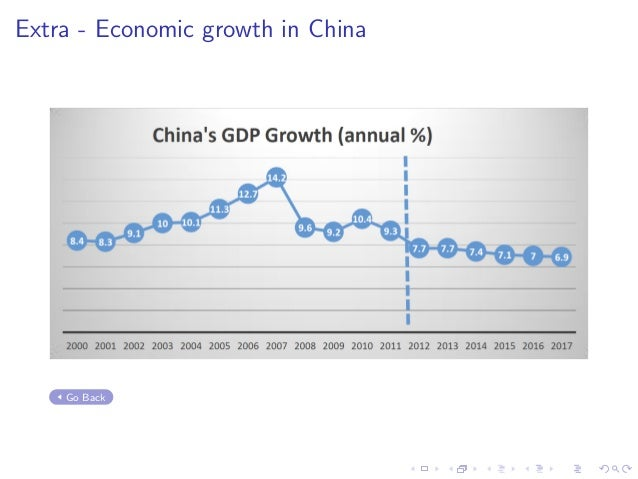Upcoming SlideShare
×

# Leniency, Asymmetric Punishment and Corruption: Preliminary Evidence from China

Presentation by Giancarlo Spagnolo "Leniency, Asymmetric Punishment and Corruption: Preliminary Evidence from China" at the SITE Corruption Conference, 31 August 2015.

Find more at: https://www.hhs.se/site

• Full Name
Comment goes here.

Are you sure you want to Yes No• Be the first to comment

• Be the first to like this

### Leniency, Asymmetric Punishment and Corruption: Preliminary Evidence from China

1. 1. Leniency, Asymmetric Punishment and Corruption Preliminary Evidence from China Maria Perrotta Berlin1 Bei Qin2 Giancarlo Spagnolo3 SITE CONFERENCE 2015 Stockholm, Aug. 31 - Sept. 1 1 SITE 2 University of Hong Kong 3 SITE, University of Rome ”Tor Vergata”, Eief, CEPR
2. 2. Three Years of Corruption Crackdown Big discussion: Biased against Xi Jinpings potential rivals? Is it the reason behind current economic slowdown? Extra Less discussed: Entering the 2nd stage, legal reinforcement Amendment IX proposed in October 2014 includes heavier penalties, but leniency and asymmetric punishment become more limited and discretional
3. 3. Leniency Deﬁnition: Immunity (or reduced sanctions) conditional on self-reporting Potentially very powerful: may deter collusion and corruption at very low cost (Spagnolo 2004; Bigoni et al, RAND 2012, JLEO 2015...) Main instrument to ﬁght collusion in antitrust since 1993 (huge literature, see Miller AER 2009; Marvao and Spagnolo, 2015) May be counterproductive if poorly designed or implemented (Buccirossi and Spagnolo, JPubEc 2006) May solve several of the problems raised by asymmetric punishment (Dufwenberg and Spagnolo, EI 2015)
4. 4. Asymmetric punishment “Why, for a Class of Bribes, the Act of Giving a Bribe Should Be Treated as Legal” — Kaushik Basu, 2011 Lots of misunderstanding of the proposal (innocent and not) Some fair criticism on its viability (e.g. by Dreze, see discussion in Dufwenberg and Spagnolo, EI 2015) Mixed experimental evidence (e.g. Abbink et al, JPubEc 2014) Xingxing Li: in China since 1997, it did not work. ...Didn’t it?
5. 5. What we do Claim diﬃcult to test as changes in total corruption (what matters) unobservable. To infer changes in total (unobserved) corruption from changes in discovered cases we adapt methodology of Miller, AER 2009: TEST: If policy improves deterrence, we should see a stable fall in the number of discovered cases after policy is introduced DATA: Number of prosecutions from the Procuratorates’ Yearly Reports, in printed version, for each of the Chinese provinces since 1986 and from the Chinese National Bureau of Statistics for 1998-2010
6. 6. Test for structural break q q q q q q q q q q q q q q q q q q q q q q q b = −16.367b = −16.367b = −16.367b = −16.367b = −16.367b = −16.367b = −16.367b = −16.367b = −16.367b = −16.367b = −16.367b = −16.367b = −16.367b = −16.367b = −16.367b = −16.367b = −16.367b = −16.367b = −16.367b = −16.367b = −16.367b = −16.367b = −16.367 t = −3.8t = −3.8t = −3.8t = −3.8t = −3.8t = −3.8t = −3.8t = −3.8t = −3.8t = −3.8t = −3.8t = −3.8t = −3.8t = −3.8t = −3.8t = −3.8t = −3.8t = −3.8t = −3.8t = −3.8t = −3.8t = −3.8t = −3.8 10 20 30 40 1988 1989 1990 1991 1992 1993 1994 1995 1996 1997 1998 1999 2000 2001 2002 2003 2004 2005 2006 2007 2008 2009 2010 Year Population−adjustednumberofcases Actualandestimated−OLS Consistent with signiﬁcant deterrence eﬀect of legal change (leniency and asymmetric punishment strengthened) in 1997 (red vertical line)
7. 7. Robustness - diﬀerent polynomials q q q q q q q q q q q q q q q q q q q q q q q q q q q q q q q q q q q q q q q q q q q q q q q q q q q q q q q q q q q q q q q q q q q q q q q q q q q q q q q q q q q q q q q q q q q q
8. 8. Robustness - Subset of provinces q q q q q q q q q q q q q q q q q q q q q q q q b = −19.1b = −19.1b = −19.1b = −19.1b = −19.1b = −19.1b = −19.1b = −19.1b = −19.1b = −19.1b = −19.1b = −19.1b = −19.1b = −19.1b = −19.1b = −19.1b = −19.1b = −19.1b = −19.1b = −19.1b = −19.1b = −19.1b = −19.1b = −19.1 t = −5.03t = −5.03t = −5.03t = −5.03t = −5.03t = −5.03t = −5.03t = −5.03t = −5.03t = −5.03t = −5.03t = −5.03t = −5.03t = −5.03t = −5.03t = −5.03t = −5.03t = −5.03t = −5.03t = −5.03t = −5.03t = −5.03t = −5.03t = −5.03 10 20 30 1988 1989 1990 1991 1992 1993 1994 1995 1996 1997 1998 1999 2000 2001 2002 2003 2004 2005 2006 2007 2008 2009 2010 Year Population−adjustednumberofcases Actualandestimated−OLS
9. 9. Caveats: much left to do VERY PRELIMINARY We don’t know which cases are extortionary (harassment) bribes and which to gain illegitimate beneﬁt We don’t know in which cases leniency was applied and in which asymmetric sanctions were For asymmetric punishments, potential denomination eﬀect if briber-givers’ and bribe-takers’ cases recorded separately (However, in Guo, 2008, 4-9% of bribery cases about bribe-givers only) Plan of case ﬁles analysis: Collect all the missing information for a sample before and after change
10. 10. Thank you
11. 11. Extra - Economic growth in China Go Back

Total views

532

On Slideshare

0

From embeds

0

Number of embeds

7

3

Shares

0